×#### Thank you for registering.

One of our academic counsellors will contact you within 1 working day.

Click to Chat

1800-1023-196

+91-120-4616500

CART 0

• 0

MY CART (5)

Use Coupon: CART20 and get 20% off on all online Study Material

ITEM
DETAILS
MRP
DISCOUNT
FINAL PRICE
Total Price: Rs.

There are no items in this cart.
Continue Shopping• Complete Physics Course - Class 11
• OFFERED PRICE: Rs. 2,968
• View Details

Work

Table of Content

Work Done by a Constant Force

Special Cases of Work Done by a Constant Force

Work Depends on the Frame of Reference

Conservative and Non-Conservative Forces

Work Done by a Variable Force

Dimension Formula for Work

Units of Work

Solved Problems

Related Resources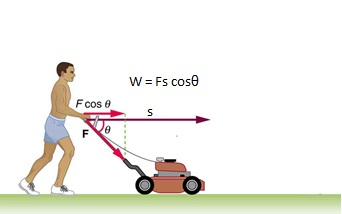Work is said to be done if a force, acting on a body, displaces the body through a certain distance and the force has some component along the displacements. Thus, work is done when the point of application of a force moves.

Work Done by a Constant Force

Consider a body experiencing a force F in a direction inclined at an angle θ with the positive direction of x-axis. Let the body be displaced from A to B through a distance s.

First Definition:-

Work done is defined as the product of force and displacement.

Let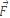be a constant force acting on a body, and let the body undergo a displacement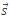, as shown in Figure given below, then the work done by the forceis defined as,W  =.=  (F cosθ)s = F(s cosθ) = Fs cosθ

Here θ is the angle between F and s.

Work done by the force is positive if the angle between force and displacement is acute (0°<θ<90°) as cos θ is positive. This signifies, when the force and displacement are in same direction, work done is positive. This work is said to be done upon the body.

Second Definition:-

Work is defined as the product of magnitude of displacement and the component of the force along the displacement.

Third Definition:-

Work is also defined as the product of magnitude of force and the component of the displacement along the direction of force.

Special Cases of Work Done by a Constant Force

Case (a):-

When θ = 0, cos θ = 1

Force and displacement are in the same direction.

So, W = Fs. (See figure given below)

Case (b):-

When, θ = 180°; cos θ = -1

Force and displacement are in opposite direction.

So, W = -Fs. (Figure given below)

Case (c)  [Zero Work]:-

When, θ = 90°; cos θ = 0

Force is perpendicular to displacement

So, W = 0 (Figure given below)

Thus, when the force acts in a direction at right angle to the direction of displacement (cos90° = 0), no work is done (zero work).Case (d) [Positive work]:-

When, 0° < θ < 90°; cos θ is positive.

Force has a component in the direction of displacement.

So, W is positive.

Case (e) [Negative Work]:-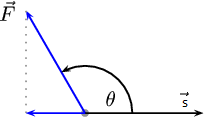When, 90° < θ < 180°, cos θ is negative

Force has a component in the direction opposite to displacement.

So, W is negative. (Figure given above)

Work done by the force is negative if the angle between force and displacement is obtuse (90°<θ<180°)  as cosθ is negative. This signifies, when the force and displacement are in opposite direction, work done is negative. This work is said to be done by the body.

Work Depends on the Frame of Reference

If a person is pushing a box inside a moving train, the work done in the frame of train will be while that in the frame of earth will be ,  where  is the displacement of the train relative to the ground.

Work done by friction may be zero, positive or negative depending upon the situation. When force applied on a body is insufficient to overcome the friction, work done by the friction force is zero. When this force is large enough to overcome the friction then, work done by the friction force is negative. When force is applied on a body that is placed above another body, the work done by the friction force on the lower body is positive.

Let us consider the situation in which a horizontal rough trolley, with a block and a man, is accelerating drawn from this.

(i)     In this case, work done by friction (between trolley and the block) is zero as observed by the man on trolley.

(ii)    Work done by friction (between trolley and the block) is positive as observed by an observer on the ground.

(iii)    Work done by friction is negative as observed by an observer who is moving along the direction of motion of trolley with higher speed.

Refer this video for better understanding about work-energy:-

Conservative and Non-Conservative Forces

There are two types of forces:

(i) conservative forces, and

(ii) non-conservative forces.

If the work done by a force is independent of the path followed, it is called a conservative force, e.g. gravitational force, electrostatic force, etc. If the work done by a force depends upon the path followed, it is called a
non-conservative force, e.g. friction, viscous force, etc.

Alternatively, work done by a conservative force in a closed loop is zero & by non-conservative forces it is not zero for a round trip.

Work Done by a Variable Force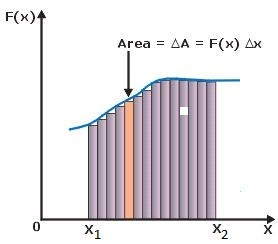Consider a body being moved, along an arbitrary path, by a force which continuously changes in magnitude as well as direction.

We first consider a force, acting on a body that varies in magnitude only. Assume that the magnitude of force is a function of position x that is F(x), acting in the positive x direction. We should like to find out the work done, by F in moving a body from X1 to X2. Here W is given by

W =The physical significance of definite integral is area under the curve.

Hence, W is nothing but Area under the curve F(x) between the lines x=x1 and x=x2 as shown in figure given below.

Now, consider that the force F changes its magnitude as well as direction from point to point. The work done by the variable force F on the body for two points on the curve a b, as the body moves from a to b will be,

Wab =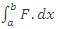This integral is called a line integral. We can evaluate this integral and obtain the work done if we know how F and θ vary from point to point.

Dimension Formula for Work

[M1 L2T-2]

Units of Work

The unit of work done in S.I is joule (J) and in C.G.S system is erg.

1J = 1 N.m , 1 erg = 1 dyn.cm

joule:- Work done is said to be one joule if a force of 1 newton displaces a body through a distance of 1 m along the direction of force.

1 J = (1 N) (1 m) = 1 kg m2s-2

erg:- Work done is said to be one erg if a force of 1 dyne displaces a body through a distance of 1 cm along the direction of force.

1 erg = (1 dyne) (1 cm) = 1 g cm2s-2

Relation between Joule and erg:- 1 J = 107 erg

Solved Problems

Problem 1:-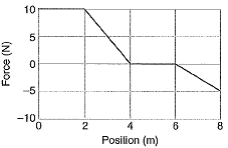A 5.0-kg block moves in a straight line on a horizontal frictionless surface under the influence of a force that varies with position as shown in below figure. How much work is done by the force as the block moves from the origin to x = 8.0 m?

Concept:-

The area under the force displacement curve is equal to the work is done by the force. So in the below figure, work is done by the force as the block moves from the origin to x = 8.0 m will be equal to the sum of area under each curve and the line F = 0.

Solution:-

Area under the first curve (A1 = W1) which is a rectangle having length 10 N and width 2 S will be,

W1 = (10 N) (2 s) = 20 N. s

= (20 N. s) (1 J/1 N. s)

= 20 J    …… (1)

Area under the second curve (A2 = W2) which is a triangle having height 10 N and base 2 S will be,

W2 = ½ (10 N) (2 s) = ½ (20 N. s)

= (10 N. s) (1 J/1 N. s)

= 10 J    …… (2)

There no area (A3 = 0) under the third curve, therefore the work done for the third curve (A3 = W3) will be zero.

W3 = 0     …… (3)

Area under the fourth curve (A4 = W4) which is a triangle having height -5N and base 2 S will be,

W4 = ½ (-5 N) (2 s) = ½ (-10 N. s)

= (-5 N. s) (1 J/1 N. s)

= -5 J    …… (4)

Thus the total work is done by the force as the block moves from the origin to x = 8.0 m will be,

W = W1+ W2 + W3+ W4

= 20 J+10 J+0+ (-5 J)

= 25 J      …… (5)

From equation (5) we observed that, work is done by the force as the block moves from the origin to x = 8.0 m will be 25 J.

Problem 2:-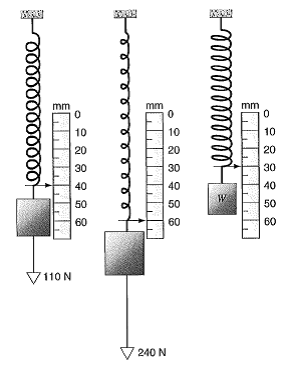Figure below shows a spring with a pointer attached, hanging next to a scale graduated in millimeters. Three different weights are hung from the spring, in turn, as shown. (a) If all weight is removed from the spring, which mark on the scale will the pointer indicate? (b) Find the weight W.

Concept:-

The restoring force F acting on the spring is defined as,

F = -kx

Here k is the force constant and x is the elongation.

So, ΔF = -kΔx

So the force constant k will be,

k = - ΔF/Δx

and

the change in elongation Δx will be,

Δx = - ΔF/k

Solution:-

(a) The force constant k will be,

k = - ΔF/ Δx

= - [(-240 N) – (-110 N)]/[60 mm-40 mm]

= 6500 N/m

Thus the change in elongation Δx with no force on the spring will be,

Δx = - ΔF/k

= -(0 N) – (-110 N)/( 6500 N/m)

= (-0.017 m) 103 mm/1 m)

= -17 mm

This is the amount less than the 40 mm mark, so the position of the spring with no force on it is 23 mm.

(b)

To obtain the weight W, first we have to find out the change in force ΔF.

Δx = -10 mm compared to the 100 N picture.

To find out ΔF, substitute -10 mm for Δx, 6500 N/m for k in the equation ΔF = -kΔx,

ΔF = -kΔx

= - (6500 N/m)(-10 mm×10-3 mm/1 m)

= - (6500 N/m)(-0.010 m)

= 65 N

The weight W of the last object will be,

W = 110 N-65 N= 45 N

From the above observation we conclude that, the weight of the last object would be 45 N.

Problem 3:-

A 47.2-kg block of ice slides down an incline 1.62 m long and 0.902 m high. A worker pushes up on the ice parallel to the incline so that its slides down at constant speed. The coefficient of kinetic friction between the ice and the incline is 0.110. Find (a) the force exerted by the worker, (b) the work done by the worker on the block of ice, and (c) the work done by gravity on the ice.

Concept:-

Weight of the block W is defined as,

W= mg

Here m is the mass of the block and g is the free fall acceleration.

Work done W on a body is equal to the applied force P on the body  times displacement x of the body.

W=Px

Solution:-(a) The below figure shows a 47.2 kg  block of ice slides down an incline 1.62 m long and 0.902 m high.

From the above figure,

sin θ = p/h

So the angle of incline θ will be,

θ = sin-1 (p/h)

= sin-1 (0.902 m/1.62 m)

= 33.8°

The parallel components of the weight is,

WParallel = mgsin θ

And the perpendicular component is,

WPerpendicular = mgcos θ

The normal force on the ice is,

N=WPerpendicular

So the frictional force f will be,

f= µk mgcosθ

Here µk is the coefficient of kinetic friction, m is the mass and g is the free fall acceleration.

The push required to allow the ice to slide down at constant speed is then found by noting that P=WParallel -f.

Then the force exerted by the worker will be,

P=WParallel -f

= mgsin θ- µk mgcosθ

= mg(sin θ- µk cosθ)

To obtain the force exerted by the worker, substitute 47.2 kg for m, 9.81 m/s2 for g, 33.8° for θ, 0.110 for µk in the equation P= mg(sin θ- µk cosθ),

P= mg(sin θ- µk cosθ)

= (47.2 kg)( 9.81 m/s2)[ sin (33.8°) - 0.110 cos(33.8°)]

=(215 kg.m/s2) (1 N/1 kg.m/s2)

=215 N

From the above observation we conclude that, the force exerted by the worker will be 215 N.

(b) To obtain the worker done by the applied force W, substitute 215 N for P and -1.62 m for x in the equation W=Px,

W=Px

=(215 N)(-1.62 m)

= (-348 N.m) (1 J/1 N.m)

= -348 J

Thus the worker done by the applied force W would be -348 J.

(c) The work done W by the force gravity will be,

W=mgx cos(90°-θ)

To obtain the work done W by the force gravity, substitute 47.2 kg for mass m, 9.81 m/s2 for g, 33.8° for θ and 1.62 m for x in the equation W=mgx cos(90°-θ),

W=mgx cos(90°-θ)

=(47.2 kg)( 9.81 m/s2)(1.62 m) cos(90°-33.8°)

=(47.2 kg)( 9.81 m/s2)(1.62 m) cos(56.2°)

=(417 kg.m2/s2) (1 J/1 kg.m2/s2)

=417 J

From the above observation we conclude that, the work done W by the force gravity will be 417 J.

Related Resources

Look into the Sample Papers of Previous Years to get a hint of the kinds of questions asked in the exam.

You can get the knowledge of Useful Books of Physics.### Course Features

• 101 Video Lectures
• Revision Notes
• Previous Year Papers
• Mind Map
• Study Planner
• NCERT Solutions
• Discussion Forum
• Test paper with Video Solution